Example Questions

1 2 3 4 5 7 Next →

Example Question #61 : How To Find Order Of Operations

Consider the expression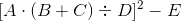If the values of all of the variables are known which operation is carried out third?

Squaring

Division

Subtraction

Multiplication

Division

Explanation:

The order of operations dictates that any operations within grouping symbols is carried out first, going from the innermost symbols to the outermost symbols. The innermost symbols - the parentheses - flank an addition, so that operation is carried out first.

What remains within the outermost symbols - the brackets - are a multiplication and a division. Between the two, the one that is at left is carried out first; this means the multiplication is performed second, then the division is worked out third.

Example Question #62 : How To Find Order Of Operations

Define an operation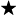on the set of real numbers as follows:

For all real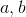,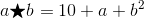Evaluate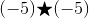.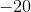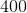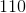Explanation:

Set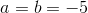in the expression in the definition, then evaluate according to the order of operations: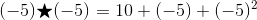Square first, remembering that the square of any number is nonnegative: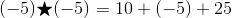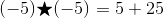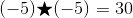Example Question #63 : How To Find Order Of Operations

Define an operationon the set of real numbers as follows:

For all real,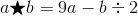Evaluate the expression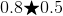.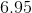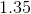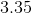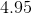None of the other responses gives a correct answer.Explanation:

Set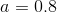and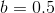in the expression in the definition, then evaluate according to the order of operations: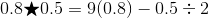Multiplication and division take precedence over subraction; carry out the multiplication first, since it is the operation at left: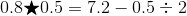Divide next: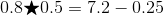Now subtract: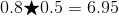Example Question #64 : How To Find Order Of Operations

Define an operationon the set of real numbers as follows:

For all real,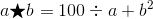Evaluate: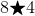.

None of the other responses gives a correct answer.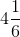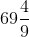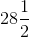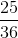Explanation:

Set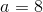and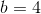in the expression in the definition, then evaluate according to the order of operations: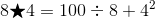By order of operations, carry out the exponentiation first: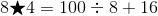The division is carried out next: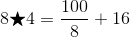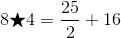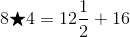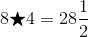Example Question #65 : How To Find Order Of Operations

Consider the expression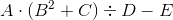If the values of all of the variables are known, which operation is carried out third?

Division

Multiplication

Squaring

Subtraction

Multiplication

Explanation:

The order of operations dictates that any operations within grouping symbols, such as parentheses, be carried out first; in this expression. There are two expressions - an exponentiation (squaring) and an addition - that fall between the parentheses; by the order of operations, the squaring is carried out, then the addition.

This leaves a multiplication, a division, and a subtraction. Multiplication and division have precedence over subtraction; since the multiplication appears first in left-to-right order, the multiplication is carried out next. This makes multiplication the correct response.

Example Question #66 : How To Find Order Of Operations

Evaluate the following expression: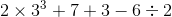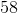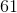Explanation:

We refer to the order of operations to determine how to properly interpret a mathematical statement involving more than one operation. In this case, the operations involved are multiplication, exponentiation, addition, subtraction, and division, as can be seen from the reproduction of the expression below:According to the order of operations, we focus our attention on the term with exponents first: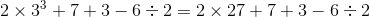Next, we deal with the multiplication and division operations from left to right: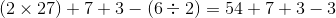Finally, we deal with the addition and subraction operations from left to right: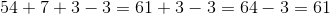Hence, the correct answer to the evaluation of the given expression is.

Example Question #67 : How To Find Order Of Operations

Which expression is equivalent to the following quotient? For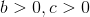.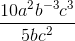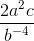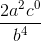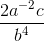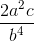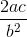Explanation:

All we need to do is remember the quotient rule for exponents.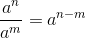We apply this to each term and we get the following.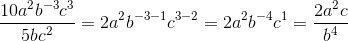Example Question #68 : How To Find Order Of Operations

25 *( 47 + 72 + 192)/12 + 65 = ?

156

87

2273

1065

172

1065

Explanation:

Order of operations: "PEMDAS” or "Please Excuse My Dear Aunt Sally"

"Parentheses, Exponents, Multiplication and Division, and Addition and Subtraction".

25 *( 47 + 72 + 192)/12 + 65 =

25 * (47 + 72 + 361) / 12 + 65 =

25 * ( 480) / 12 + 65 =

12000 / 12 + 65 =

1000 + 65 = 1065

Example Question #69 : How To Find Order Of Operations

Find the Ratio of Solution 1 to Solution 2 in the following quadratic equation, where Solution 1 is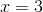and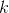is a constant.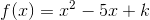Explanation:

The first step is to solve for, so we will plug in the solution.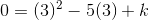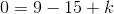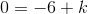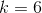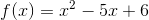Since we know one solution, we can do synthetic division to figure out the other solution.

We can guess and check for the other solution which is, so in equation form we have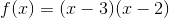The ratio is then.

Example Question #70 : How To Find Order Of Operations

(28 / 14) 3  + ( 144 – 12 )*17 + 362 = ?

22032

2288

2452

1652

3548

3548

Explanation:

Order of operations: "PEMDAS” or "Please Excuse My Dear Aunt Sally"

"Parentheses, Exponents, Multiplication and Division, and Addition and Subtraction".

(28 / 14) 3  + ( 144 – 12 )*17 + 362 =

(2) 3  + ( 132 )*17 + 362 =

8 + ( 132 )*17 + 1296 =

8 + 2244 + 1296 =

3548

1 2 3 4 5 7 Next →